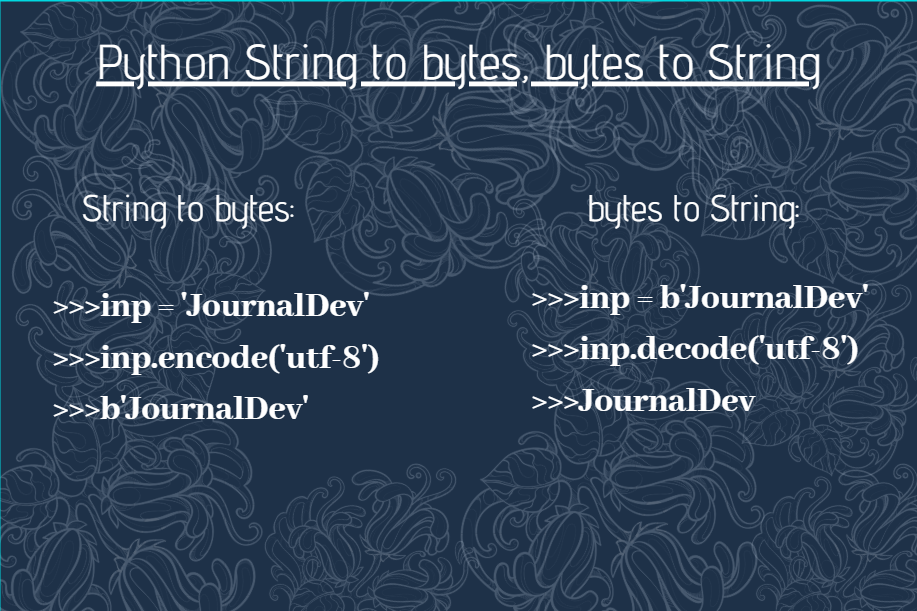# Python String to bytes, bytes to StringIn this article, we will have a look at the conversion of Python String to bytes and Python bytes to String. Python conversion of type has gained quite an importance due to its feature of data being used during various operations in a different form.

Python conversion of String to bytes and bytes to String has its own importance for the fact that it is necessary while file handling, etc.

## Python String to bytes

Either of the following ways can be used to convert Python String to bytes:

• Using `bytes()` method
• Using `encode()` method

### 1. Python String to bytes using bytes() method

Python’s CPython library provides us with `bytes()` function to convert String to bytes.

Syntax:

```bytes(input_string, 'utf-8')
```

Note: The UTF-8 format is used for the purpose of encoding.

Example:

```inp = "Engineering Discipline"

print("Input String:\n")
print(str(inp))

opt = bytes(inp, 'utf-8')

print("String after getting converted to bytes:\n")
print(str(opt))
print(str(type(opt)))
```

Output:

```Input String:

Engineering Discipline
String after getting converted to bytes:

b'Engineering Discipline'
<class 'bytes'>

```

### 2. Python String to bytes using encode() method

Python’s `encode()` method can also be used to convert a String to byte format.

Syntax:

```input_string.encode('utf-8')
```

Example:

```inp = "Engineering Discipline"

print("Input String:\n")
print(str(inp))

opt = inp.encode('utf-8')

print("String after getting converted to bytes:\n")
print(str(opt))
print(str(type(opt)))

```

Output:

```Input String:

Engineering Discipline
String after getting converted to bytes:

b'Engineering Discipline'
<class 'bytes'>

```

## Python bytes to String

Python’s byte class has built-in `decode()` method to convert Python bytes to String.

Syntax:

```string.decode('utf-8')
```

Example:

```inp = "Engineering Discipline"

print("Input String:\n")
print(str(inp))

opt = inp.encode('utf-8')

print("String after getting converted to bytes:\n")
print(str(opt))
print(str(type(opt)))

original = opt.decode('utf-8')
print("The decoded String i.e. byte to converted string:\n")
print(str(original))

```

In the above example, we have initially converted the input string to bytes using the encode() method. After which, the decode() method converts that encoded input to original string.

Output:

```Input String:

Engineering Discipline
String after getting converted to bytes:

b'Engineering Discipline'
<class 'bytes'>
The decoded String i.e. byte to converted string:

Engineering Discipline
```

### Pandas bytes to String

Pandas module has got `Series.str.decode()` method to convert the encoded data i.e. the data in bytes format to String format.

Syntax:

```input_string.decode(encoding = 'UTF-8')
```

Example:

```import pandas

inp = pandas.Series([b"b'Jim'", b"b'Jonny'", b"b'Shawn'"])

print("Encoded String:")
print(inp)

opt = inp.str.decode(encoding = 'UTF-8')
print("\n")
print("Decoded String:")
print(opt)

```

In the above example, we assume the data to be in encoded format. Further which, manipulations are performed on the data.

Output:

```Encoded String:
0    b"b'Jim'"
1    b"b'Jonny'"
2    b"b'Shawn'"
dtype: object

Decoded String:
0    b'Jim'
1    b'Jonny'
2    b'Shawn'
dtype: object
​
```

## Conclusion

In this article, we have understood the conversion of Python String to bytes and vice versa which also ponders over the concept of encoding and decoding.

## References

Python String to bytes, bytes to String – JournalDev